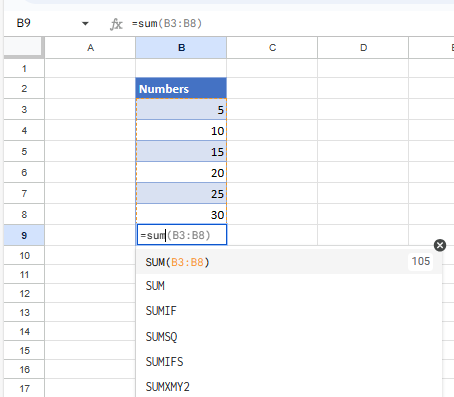# How to Use Formula AutoComplete in Excel & Google Sheets

This tutorial demonstrates how to use formula autocomplete in Excel and Google Sheets.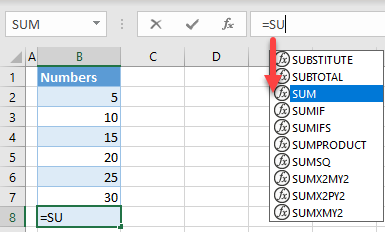## Use Formula AutoComplete

When inserting formula in Excel, you can type the first few letters of the function in the formula bar and use the suggestion to autocomplete the formula.

1. Say you want to use the SUM Function, to sum values from cells B2:B7, in cell B8. Select cell B2, and type =SU. You get a list of functions starting with SU–.
Tip: You could also start typing the function (=SU) directly into a cell instead of the formula bar.1. Now navigate with the (down arrow) key until the SUM Function is highlighted. Then press TAB on your keyboard to autocomplete the function name.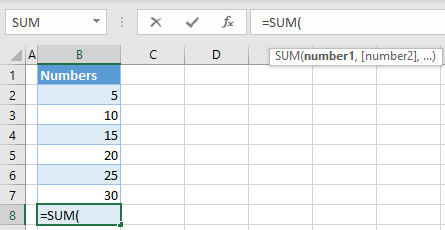1. Now that you have the function, you can also see input parameters. Select the range of cells to add up (B2:B7), close the parentheses and press ENTER.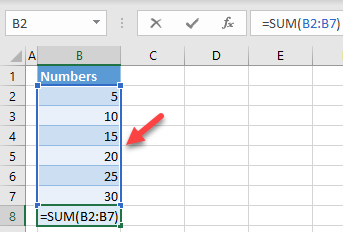Finally, you have the complete function in cell B8.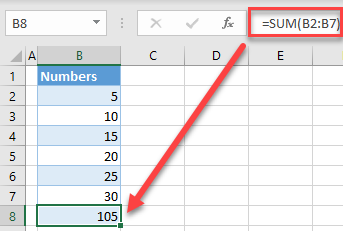## Autocomplete Formula in Google Sheets